# Dividing polynomials by binomials

 To divide polynomials by binomials, we must use long division. This process looks confusing at first, but once you get the hang of it, it's actually pretty easy. The steps match the steps you take to do a long division problem with numbers: 1) Divide 2) Multiply 3) Subtract 4) Bring Down 5) Repeat Let's go through an example step-by-step to see how this works: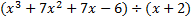First, we will write this as a long division problem: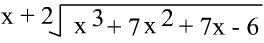Now we will follow the steps above. Step1:Divide.We will divided the first term of the polynomial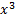by x.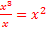,so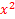goes at the top as part of our answer:Step 2: Multiply. We multiply the piece we just put as part of the answer () by the entire binomial (ð¥+2).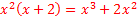.This is written underneath the original polynomial (just like we would in an arithmetic long division problem0.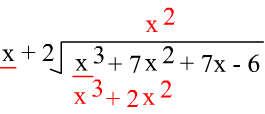Step 3: Subtract. We now need to subtract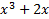.Remember that to subtract a polynomial you have to change the sign of each term then combine like terms as shown here: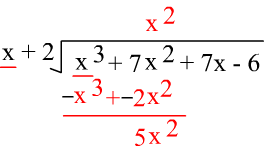Step 4: Bring down. Simply bring down the next term in the polynomial: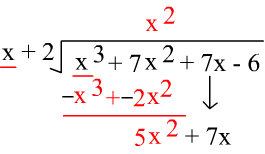Step 5: Repeat. Now we start back again at the beginning with division Divide: Now we will divide the first term of our answer 5x2 by x.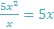, so 5x goes at the top as part of our answer: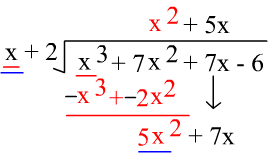Multiply: Now we multiply the piece we just put as part of the answer (5x) by the entire binomial (x+2).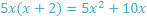.This is written underneath (just like we would in an arithmetic long division problem).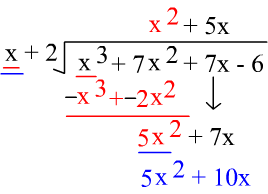Subtract: We now need to subtract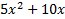.Remember that to subtract a polynomial you have to change the sign of each term then combine like terms as shown here: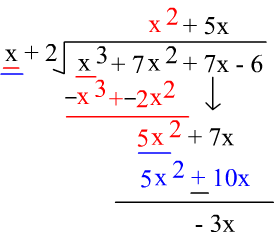Bring down: Simply bring down the next term in the polynomial: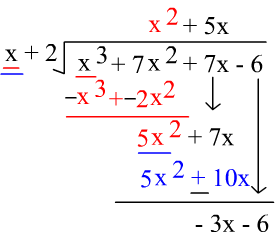Repeat: Once again we start back at the beginning with division Divide: Now we will divide the first term of our answer -3x by x.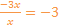, so -3 goes at the top as part of our answer: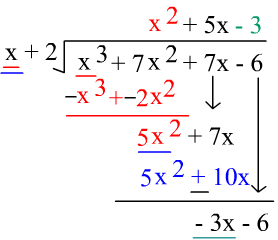Multiply: Now we multiply the piece we just put as part of the answer (-3) by the entire binomial (x+2).-3(x + 2) = -3x -6.This is written underneath the original polynomial (just like we would in an arithmetic long division problem.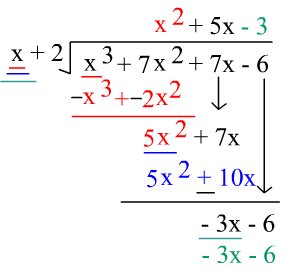Step 6: Subtract. We now need to subtract -3x-6.. Remember that to subtract a polynomial you have to change the sign of each term then combine like terms as shown here: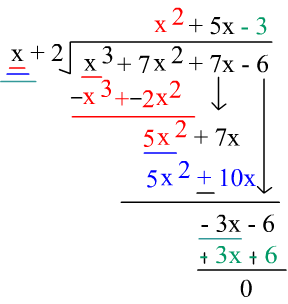We have no remainder, so we are finished, and our answer is the trinomial at the top: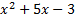As you can see, there are lots of steps to these problems, but the steps are repetitive, so once you understand the pattern, you can solve them without too much trouble. Take a few minutes to read through the steps above again, working the problem for yourself on a sheet of paper as you go. Now, try to solve this problem on your own: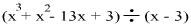. For help, look through the steps above and work through each step of the new problem piece by piece. Here's how this problem looks worked out. If you didn't get the right answer, check your work to see where you went wrong.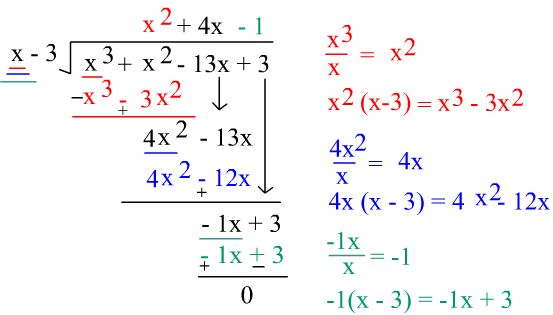Practice: Divide the following 1)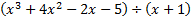2)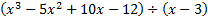3)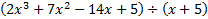Answers: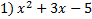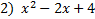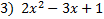Related Links: Math Algebra Polynomials Algebra Topics